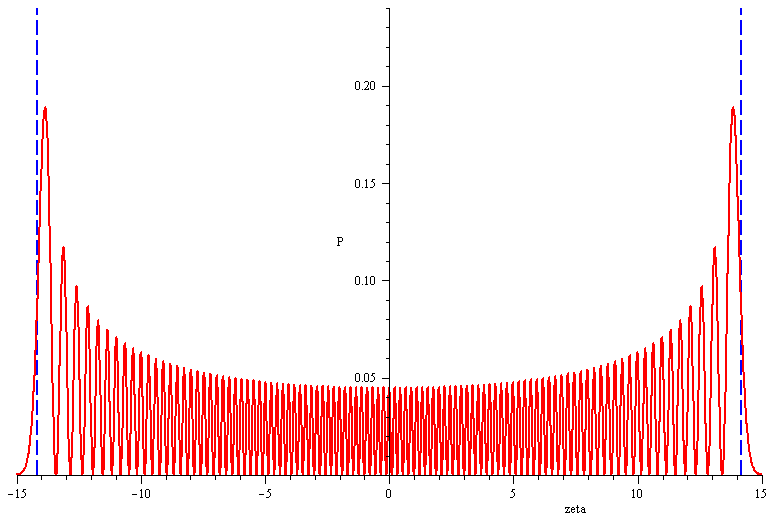# Quantum Harmonic Oscillator: Power series method in Maple

Standard

In the previous blog post What is Computational Physics (Science)?, I ended the post with the following figureGraph of the probability distribution of the 100th state of the quantum harmonic oscillator (generated using the power series method).

and stated that I might write a post on how to solve the Quantum harmonic oscillator numerically using the power series method (the other method being the ladder operator method ) and generate that figure. This post is just about that.

Ok. First I need to clear the cache with the restart command, import the PDEtools (to solve the pde SE) and Maplets[Elements] (necessary if you want to generate a maplet with a slider) packages.

```restart;
with(PDEtools): #we need to use the dchange command later in the solution
with(Maplets[Elements]):```